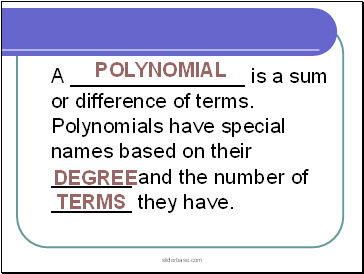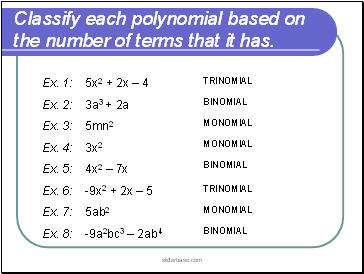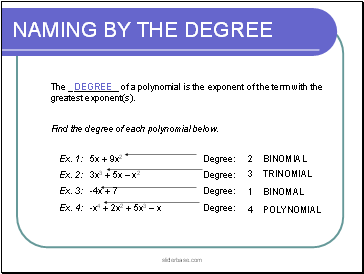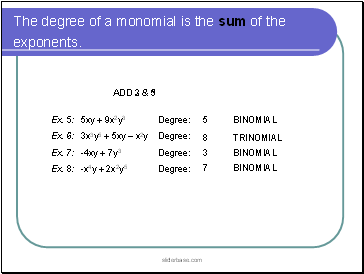# Classifying PolynomialsPage 1

#### WATCH ALL SLIDES

Slide 1CLASSIFYING POLYNOMIALS

Slide 2A _ is a sum or difference of terms. Polynomials have special names based on their _ and the number of _ they have.

POLYNOMIAL

DEGREE

TERMS

Slide 3## NAMING BY NUMBER OF TERMS

POLYNOMIALS

MONOMIALS

(1 TERM)

BINOMIALS

(2 TERMS)

TRINOMIALS

(3 TERMS)

Slide 4Classify each polynomial based on the number of terms that it has.

Ex. 1: 5x2 + 2x – 4

Ex. 2: 3a3 + 2a

Ex. 3: 5mn2

Ex. 4: 3x2

Ex. 5: 4x2 – 7x

Ex. 6: -9x2 + 2x – 5

Ex. 7: 5ab2

Ex. 8: -9a2bc3 – 2ab4

TRINOMIAL

BINOMIAL

MONOMIAL

MONOMIAL

BINOMIAL

TRINOMIAL

MONOMIAL

BINOMIAL

Slide 5## NAMING BY THE DEGREE

The of a polynomial is the exponent of the term with the greatest exponent(s).

DEGREE

Find the degree of each polynomial below.

Ex. 1: 5x + 9x2 Degree:

Ex. 2: 3x3 + 5x – x2 Degree:

Ex. 3: -4x + 7 Degree:

Ex. 4: -x4 + 2x2 + 5x3 – x Degree:

2

3

1

4

BINOMIAL

TRINOMIAL

BINOMAL

POLYNOMIAL

Slide 6The degree of a monomial is the sum of the exponents.

Ex. 5: 5xy + 9x2y3 Degree:

Ex. 6: 3x3y5 + 5xy – x2y Degree:

Ex. 7: -4xy + 7y3 Degree:

Ex. 8: -x4y + 2x2y5 Degree:

5

8

3

7

BINOMIAL

TRINOMIAL

BINOMIAL

BINOMIAL

Slide 7Classify each polynomial above using its degree and number of terms.

Ex. 1

Ex. 2

Ex. 3

Ex. 4

Ex. 5

Ex. 6

Ex. 7

Ex. 8

CUBIC TRINOMIAL

LINEAR BINOMIAL

QUARTIC POLYNOMIAL

5TH DEGREE BINOMIAL

8TH DEGREE TRINOMIAL

CUBIC BINOMIAL

7TH DEGREE BINOMIAL